# Engineering Graphics I UnitI Prof Ravindra R Paliwal

• Slides: 64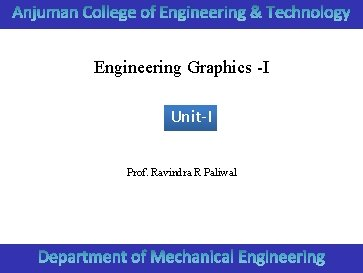Engineering Graphics -I Unit-I Prof. Ravindra R PaliwalDivide a line in n equal segments.Divide a line in n equal segments.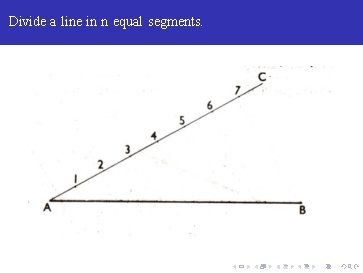Divide a line in n equal segments.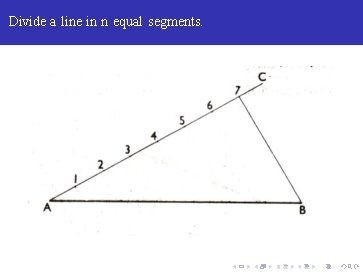Divide a line in n equal segments.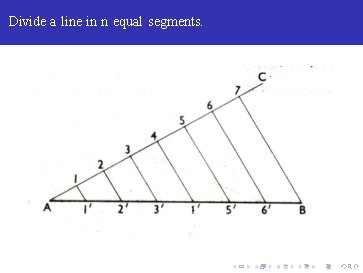Divide a line in n equal segments.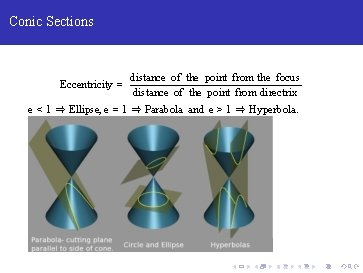Conic Sections Eccentricity = distance of the point from the focus distance of the point from directrix e < 1 ⇒ Ellipse, e = 1 ⇒ Parabola and e > 1 ⇒ Hyperbola.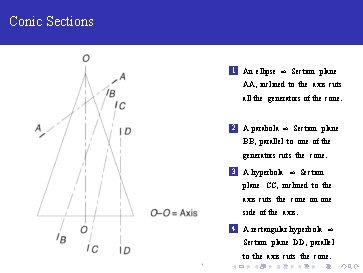Conic Sections 1 An ellipse ⇒ Section plane AA, inclined to the axis cuts all the generators of the cone. 2 A parabola ⇒ Section plane BB, parallel to one of the generators cuts the cone. 3 A hyperbola ⇒ Section plane CC, inclined to the axis cuts the cone on one side of the axis. 4 A rectangular hyperbola ⇒ Section plane DD, parallel to the axis cuts the cone.Ellipse by Directrix and Focus e = 2/3 and focus distance from directrix is known. 1 Divide CF in five equal segments. Draw VE ⊥ CF and VF = VE.Ellipse by Directrix and Focus e = 2/3 and focus distance from directrix is known. 1 Divide CF in five equal segments. Draw VE ⊥ CF and VF = VE. 2 Extend CE to cover the horizontal span of the ellipse.Ellipse by Directrix and Focus e = 2/3 and focus distance from directrix is known. 1 Divide CF in five equal segments. Draw VE ⊥ CF and VF = VE. 2 Extend CE to cover the horizontal span of the ellipse. 3 Draw a vertical at 1.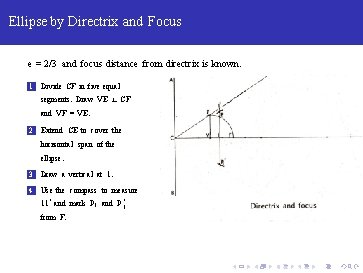Ellipse by Directrix and Focus e = 2/3 and focus distance from directrix is known. 1 Divide CF in five equal segments. Draw VE ⊥ CF and VF = VE. 2 Extend CE to cover the horizontal span of the ellipse. 3 Draw a vertical at 1. 4 Use the compass to measure 11‘ and mark P 1 and P 1‘ from F.Ellipse by Directrix and Focus e = 2/3 and focus distance from directrix is known. 1 Divide CF in five equal segments. Draw VE ⊥ CF and VF = VE. 2 Extend CE to cover the horizontal span of the ellipse. 3 Draw a vertical at 1. 4 Use the compass to measure 11‘ and mark P 1 and P 1‘ from F. Any point, P 2 or P 20 , is C 2 distance apart from the directrix and FP 2 or FP 20 apart from the focus.Ellipse by Directrix and Focus e = 2/3 and focus distance from directrix is known. 1 Divide CF in five equal segments. Draw VE ⊥ CF and VF = VE. 2 Extend CE to cover the horizontal span of the ellipse. 3 Draw a vertical at 1. 4 Use the compass to measure 11‘ and mark P 1 and P 1‘ from F. Any point, P 2 or P 20 , is C 2 distance apart from the directrix and FP 2 or FP 20 apart from the focus.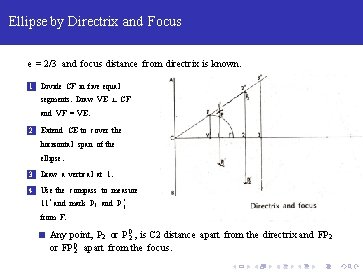Ellipse by Directrix and Focus e = 2/3 and focus distance from directrix is known. 1 Divide CF in five equal segments. Draw VE ⊥ CF and VF = VE. 2 Extend CE to cover the horizontal span of the ellipse. 3 Draw a vertical at 1. 4 Use the compass to measure 11‘ and mark P 1 and P 1‘ from F. Any point, P 2 or P 20 , is C 2 distance apart from the directrix and FP 2 or FP 20 apart from the focus.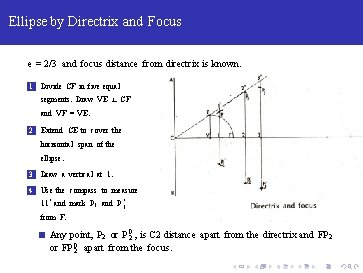Ellipse by Directrix and Focus e = 2/3 and focus distance from directrix is known. 1 Divide CF in five equal segments. Draw VE ⊥ CF and VF = VE. 2 Extend CE to cover the horizontal span of the ellipse. 3 Draw a vertical at 1. 4 Use the compass to measure 11‘ and mark P 1 and P 1‘ from F. Any point, P 2 or P 20 , is C 2 distance apart from the directrix and FP 2 or FP 20 apart from the focus.Ellipse by Directrix and Focus e = 2/3 and focus distance from directrix is known. 1 Divide CF in five equal segments. Draw VE ⊥ CF and VF = VE. 2 Extend CE to cover the horizontal span of the ellipse. 3 Draw a vertical at 1. 4 Use the compass to measure 11‘ and mark P 1 and P 1‘ from F. Any point, P 2 or P 20 , is C 2 distance apart from the directrix and FP 2 or FP 20 apart from the focus.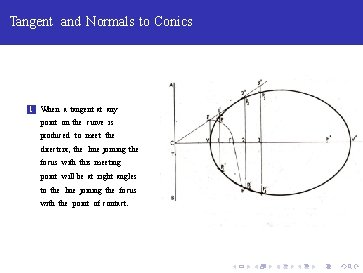Tangent and Normals to Conics 1 When a tangent at any point on the curve is produced to meet the directrix, the line joining the focus with this meeting point will be at right angles to the line joining the focus with the point of contact.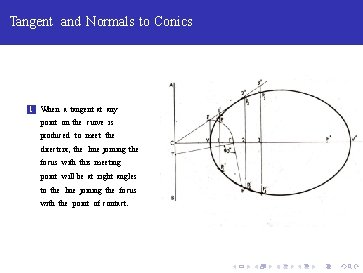Tangent and Normals to Conics 1 When a tangent at any point on the curve is produced to meet the directrix, the line joining the focus with this meeting point will be at right angles to the line joining the focus with the point of contact.Tangent and Normals to Conics 1 When a tangent at any point on the curve is produced to meet the directrix, the line joining the focus with this meeting point will be at right angles to the line joining the focus with the point of contact.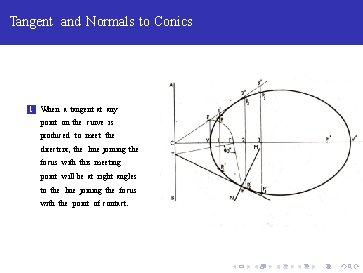Tangent and Normals to Conics 1 When a tangent at any point on the curve is produced to meet the directrix, the line joining the focus with this meeting point will be at right angles to the line joining the focus with the point of contact.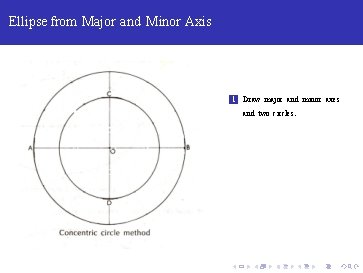Ellipse from Major and Minor Axis 1 Draw major and minor axes and two circles.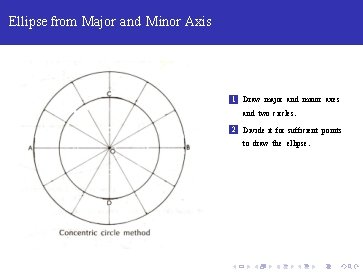Ellipse from Major and Minor Axis 1 Draw major and minor axes and two circles. 2 Divide it for sufficient points to draw the ellipse.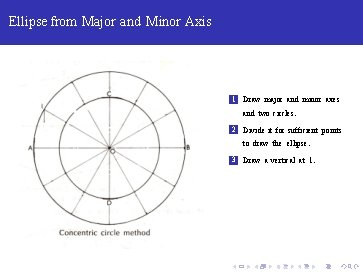Ellipse from Major and Minor Axis 1 Draw major and minor axes and two circles. 2 Divide it for sufficient points to draw the ellipse. 3 Draw a vertical at 1.Ellipse from Major and Minor Axis 1 Draw major and minor axes and two circles. 2 Divide it for sufficient points to draw the ellipse. 3 Draw a vertical at 1. 4 Draw horizontal at 1‘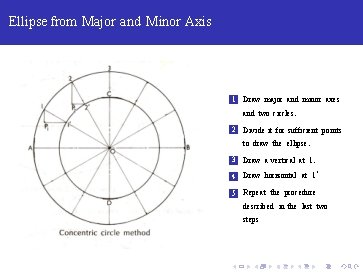Ellipse from Major and Minor Axis 1 Draw major and minor axes and two circles. 2 Divide it for sufficient points to draw the ellipse. 3 Draw a vertical at 1. 4 Draw horizontal at 1‘ 5 Repeat the procedure described in the last two stepsEllipse from Major and Minor Axis 1 Draw major and minor axes and two circles. 2 Divide it for sufficient points to draw the ellipse. 3 Draw a vertical at 1. 4 Draw horizontal at 1‘ 5 Repeat the procedure described in the last two steps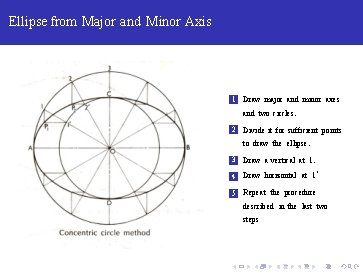Ellipse from Major and Minor Axis 1 Draw major and minor axes and two circles. 2 Divide it for sufficient points to draw the ellipse. 3 Draw a vertical at 1. 4 Draw horizontal at 1‘ 5 Repeat the procedure described in the last two steps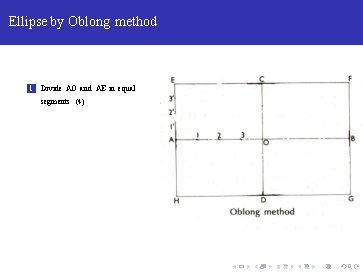Ellipse by Oblong method 1 Divide AO and AE in equal segments (4)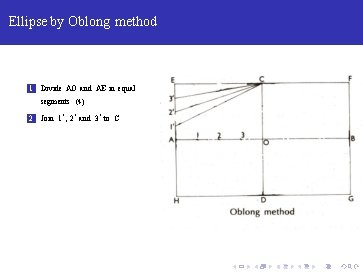Ellipse by Oblong method 1 Divide AO and AE in equal segments (4) 2 Join 1‘ , 2‘ and 3‘ to CEllipse by Oblong method 1 Divide AO and AE in equal segments (4) 2 Join 1‘ , 2‘ and 3‘ to C 3 Join 1, 2 and 3 with D and extendEllipse by Oblong method 1 Divide AO and AE in equal segments (4) 2 Join 1‘ , 2‘ and 3‘ to C 3 Join 1, 2 and 3 with D and extend 4 On vertical P 2 P 11 cut P 2 x = x. P 11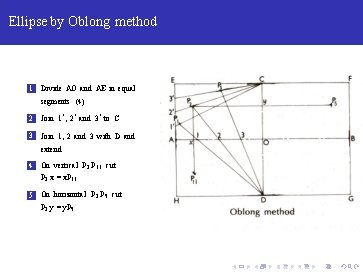Ellipse by Oblong method 1 Divide AO and AE in equal segments (4) 2 Join 1‘ , 2‘ and 3‘ to C 3 Join 1, 2 and 3 with D and extend 4 On vertical P 2 P 11 cut P 2 x = x. P 11 5 On horizontal P 2 P 5 cut P 2 y = y. P 5Ellipse by Oblong method 1 Divide AO and AE in equal segments (4) 2 Join 1‘ , 2‘ and 3‘ to C 3 Join 1, 2 and 3 with D and extend 4 On vertical P 2 P 11 cut P 2 x = x. P 11 5 On horizontal P 2 P 5 cut P 2 y = y. P 5 6 Find other point similarly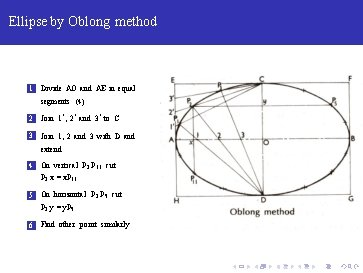Ellipse by Oblong method 1 Divide AO and AE in equal segments (4) 2 Join 1‘ , 2‘ and 3‘ to C 3 Join 1, 2 and 3 with D and extend 4 On vertical P 2 P 11 cut P 2 x = x. P 11 5 On horizontal P 2 P 5 cut P 2 y = y. P 5 6 Find other point similarly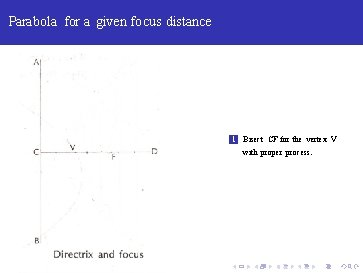Parabola for a given focus distance 1 Bisect CF for the vertex V with proper process.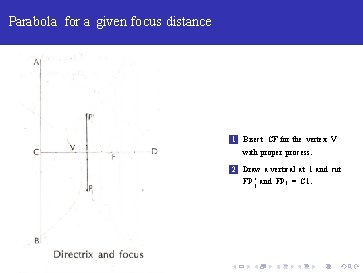Parabola for a given focus distance 1 Bisect CF for the vertex V with proper process. 2 Draw a vertical at 1 and cut FP 1‘ and FP 1 = C 1.Parabola for a given focus distance 1 Bisect CF for the vertex V with proper process. 2 Draw a vertical at 1 and cut FP 1‘ and FP 1 = C 1. 3 Repeat for more points.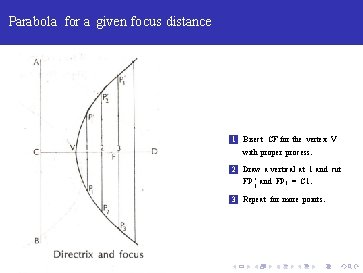Parabola for a given focus distance 1 Bisect CF for the vertex V with proper process. 2 Draw a vertical at 1 and cut FP 1‘ and FP 1 = C 1. 3 Repeat for more points.Parabola for a given base and axis 1 Draw the base AB and axis EF from mid point E.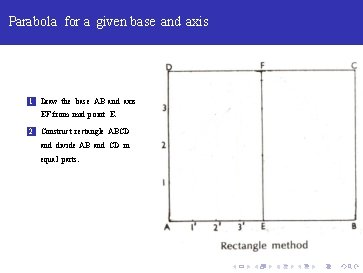Parabola for a given base and axis 1 Draw the base AB and axis EF from mid point E. 2 Construct rectangle ABCD and divide AB and CD in equal parts.Parabola for a given base and axis 1 Draw the base AB and axis EF from mid point E. 2 Construct rectangle ABCD and divide AB and CD in equal parts. 3 Draw lines F 1, F 2, F 3 and perpendiculars 1‘ P 1 , 2‘ P 2 and 3‘ P 3.Parabola for a given base and axis 1 Draw the base AB and axis EF from mid point E. 2 Construct rectangle ABCD and divide AB and CD in equal parts. 3 Draw lines F 1, F 2, F 3 and perpendiculars 1‘ P 1 , 2‘ P 2 and 3‘ P 3. 4 Cut points like P 1‘. .Parabola for a given base and axis 1 Draw the base AB and axis EF from mid point E. 2 Construct rectangle ABCD and divide AB and CD in equal parts. 3 Draw lines F 1, F 2, F 3 and perpendiculars 1‘ P 1 , 2‘ P 2 and 3‘ P 3. 4 Cut points like P 1‘. .Parabola in Parrallelogram Follow similar process.Hyperbola for a given eccentricity Follow similar process.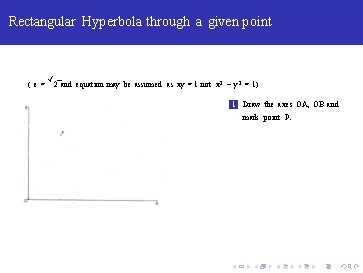Rectangular Hyperbola through a given point ( e= √ 2 and equation may be assumed as xy = 1 not x 2 − y 2 = 1) 1 Draw the axes OA, OB and mark point P.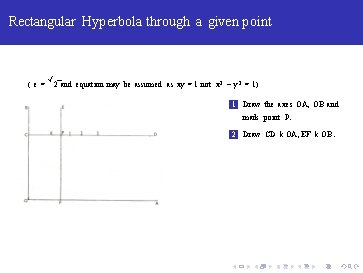Rectangular Hyperbola through a given point ( e= √ 2 and equation may be assumed as xy = 1 not x 2 − y 2 = 1) 1 Draw the axes OA, OB and mark point P. 2 Draw CD k OA, EF k OB.Rectangular Hyperbola through a given point ( e= √ 2 and equation may be assumed as xy = 1 not x 2 − y 2 = 1) 1 Draw the axes OA, OB and mark point P. 2 Draw CD k OA, EF k OB. 3 Mark points 1, 2, 3. . . (may not be equidistant)Rectangular Hyperbola through a given point ( e= √ 2 and equation may be assumed as xy = 1 not x 2 − y 2 = 1) 1 Draw the axes OA, OB and mark point P. 2 Draw CD k OA, EF k OB. 3 Mark points 1, 2, 3. . . (may not be equidistant) 4 Join O 1 and 1 P 1 k OB and 1‘ P 1 k OA to find P 1Rectangular Hyperbola through a given point ( e= √ 2 and equation may be assumed as xy = 1 not x 2 − y 2 = 1) 1 Draw the axes OA, OB and mark point P. 2 Draw CD k OA, EF k OB. 3 Mark points 1, 2, 3. . . (may not be equidistant) 4 Join O 1 and 1 P 1 k OB and 1‘ P 1 k OA to find P 1 5 Find the other points similarly.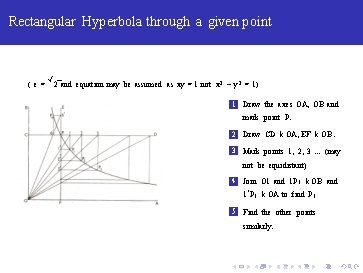Rectangular Hyperbola through a given point ( e= √ 2 and equation may be assumed as xy = 1 not x 2 − y 2 = 1) 1 Draw the axes OA, OB and mark point P. 2 Draw CD k OA, EF k OB. 3 Mark points 1, 2, 3. . . (may not be equidistant) 4 Join O 1 and 1 P 1 k OB and 1‘ P 1 k OA to find P 1 5 Find the other points similarly.Length of an Arc Length of an arc subtending an angle less than 600 and length of circumference.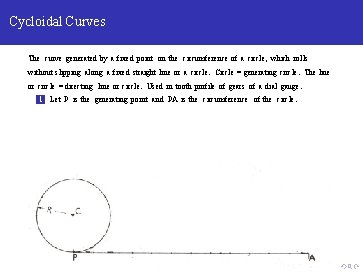Cycloidal Curves The curve generated by a fixed point on the circumference of a circle, which rolls without slipping along a fixed straight line or a circle. Circle = generating circle. The line or circle = directing line or circle. Used in tooth profile of gears of a dial gauge. 1 Let P is the generating point and PA is the circumference of the circle.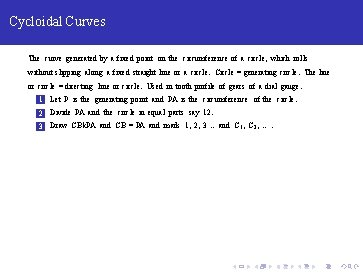Cycloidal Curves The curve generated by a fixed point on the circumference of a circle, which rolls without slipping along a fixed straight line or a circle. Circle = generating circle. The line or circle = directing line or circle. Used in tooth profile of gears of a dial gauge. 1 Let P is the generating point and PA is the circumference of the circle. 2 Divide PA and the circle in equal parts say 12. 3 Draw CBk. PA and CB = PA and mark 1, 2, 3. . and C 1 , C 2 , . . .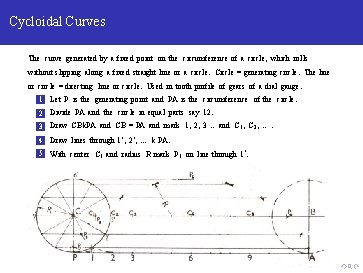Cycloidal Curves The curve generated by a fixed point on the circumference of a circle, which rolls without slipping along a fixed straight line or a circle. Circle = generating circle. The line or circle = directing line or circle. Used in tooth profile of gears of a dial gauge. 1 Let P is the generating point and PA is the circumference of the circle. 2 Divide PA and the circle in equal parts say 12. 3 Draw CBk. PA and CB = PA and mark 1, 2, 3. . and C 1 , C 2 , . . . 4 Draw lines through 1‘ , 2‘ , . . . k PA. 5 With center C 1 and radius R mark P 1 on line through 1‘.Cycloidal Curves The curve generated by a fixed point on the circumference of a circle, which rolls without slipping along a fixed straight line or a circle. Circle = generating circle. The line or circle = directing line or circle. Used in tooth profile of gears of a dial gauge. 1 Let P is the generating point and PA is the circumference of the circle. 2 Divide PA and the circle in equal parts say 12. 3 Draw CBk. PA and CB = PA and mark 1, 2, 3. . and C 1 , C 2 , . . . 4 Draw lines through 1‘ , 2‘ , . . . k PA. 5 With center C 1 and radius R mark P 1 on line through 1‘.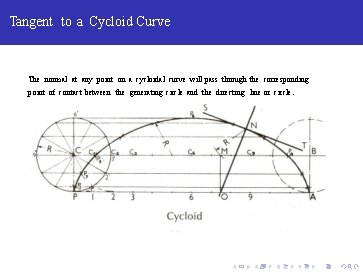Tangent to a Cycloid Curve The normal at any point on a cycloidal curve will pass through the corresponding point of contact between the generating circle and the directing line or circle.Trochoid Curves curve generated by a point fixed to a circle, within or outside its circumference, as the circle rolls along a straight line. Point within the circle = inferior trochoid, Point outside the circle = superior trochoid, 1 Process is similar to the previous. We need to extend the lines C 1 P 1 , C 2 P 2 , . . and cut the appropriate lengths like radius R 1 or R 2 from the lines.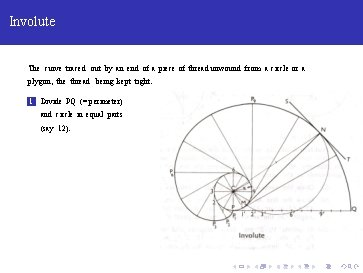Involute The curve traced out by an end of a piece of thread unwound from a circle or a plygon, the thread being kept tight. 1 Divide PQ (= perimeter) and circle in equal parts (say 12).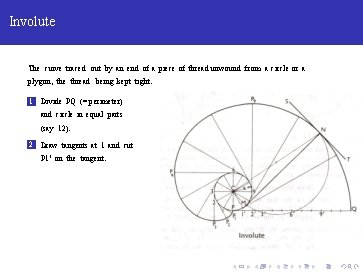Involute The curve traced out by an end of a piece of thread unwound from a circle or a plygon, the thread being kept tight. 1 Divide PQ (= perimeter) and circle in equal parts (say 12). 2 Draw tangents at 1 and cut P 1’ on the tangent.Involute The curve traced out by an end of a piece of thread unwound from a circle or a plygon, the thread being kept tight. 1 Divide PQ (= perimeter) and circle in equal parts (say 12). 2 Draw tangents at 1 and cut P 1’ on the tangent. 3 Repeat for further points.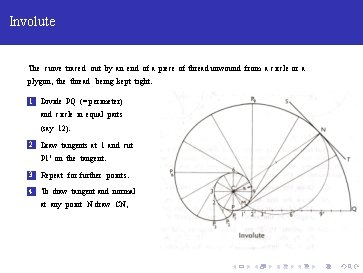Involute The curve traced out by an end of a piece of thread unwound from a circle or a plygon, the thread being kept tight. 1 Divide PQ (= perimeter) and circle in equal parts (say 12). 2 Draw tangents at 1 and cut P 1’ on the tangent. 3 Repeat for further points. 4 To draw tangent and normal at any point N draw CN,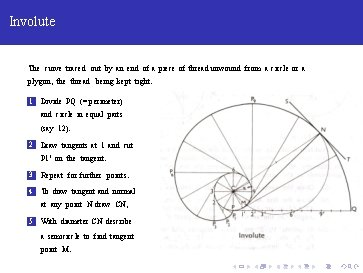Involute The curve traced out by an end of a piece of thread unwound from a circle or a plygon, the thread being kept tight. 1 Divide PQ (= perimeter) and circle in equal parts (say 12). 2 Draw tangents at 1 and cut P 1’ on the tangent. 3 Repeat for further points. 4 To draw tangent and normal at any point N draw CN, 5 With diameter CN describe a semicircle to find tangent point M.